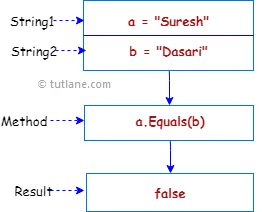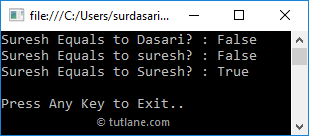# C# String Equals Method

In c#, the string Equals method is used to check whether the specified two string objects have the same value or not. If both string object values are equal, then the Equals() method will return true otherwise false.

If both string objects have a null value, then the string Equals() method will return true.

Following is the pictorial representation of using the string Equals() method to check whether the given string objects are equal or not in the c# programming language.If you observe the above diagram, we defined two strings “Suresh”, “Dasari” and checking whether both strings are equal or not using the Equals method.

## C# String Equals Method Syntax

Following is the syntax of defining a string Equals method to check whether the given string objects are equal or not in the c# programming language.

public bool Equals(string a, string b)

If you observe syntax, we will use both defined strings to check whether both strings are equal or not using the Equals method.

## C# String Equals Method Example

Following is the example of using the string Equals() method to check whether the given strings are equal or not in the c# programming language.

using System;

namespace Tutlane
{
class Program
{
static void Main(string[] args)
{
string fname = "Suresh";
string lname = "Dasari";
Console.WriteLine("{0} Equals to {1}? : {2}", fname, lname, fname.Equals(lname));
string l_name = "suresh";
Console.WriteLine("{0} Equals to {1}? : {2}", fname, l_name, fname.Equals(l_name));
string u_name = "Suresh";
Console.WriteLine("{0} Equals to {1}? : {2}", fname, u_name, fname.Equals(u_name));
Console.WriteLine("\nPress Enter Key to Exit..");
}
}
}

If you observe the above example, we used a string Equals() method to check whether the specified string values are equal or not.

When you execute the above c# program, we will get the result below.If you observe the above result, the string Equals() method checking the specified string values are equal or not and returned a boolean value.

## C# String Equals Ignore Case

Generally, in c# the string Equals() method will perform case-sensitive string comparison. If we want to perform case insensitive string comparison, we need to use the OrdinalIgnoreCase property and the Equals method.

Following is the example of ignoring the case while comparing the strings in the c# programming language.

using System;

namespace Tutlane
{
class Program
{
static void Main(string[] args)
{
string fname = "Suresh";
string l_name = "suresh";
Console.WriteLine("{0} Equals to {1}? : {2}", fname, l_name, fname.Equals(l_name, StringComparison.OrdinalIgnoreCase));
string u_name = "Suresh";
Console.WriteLine("{0} Equals to {1}? : {2}", fname, u_name, fname.Equals(u_name));
Console.WriteLine("\nPress Enter Key to Exit..");
}
}
}

If you observe the above example, we used an “OrdinalIgnoreCase” property with Equals() method to perform case insensitive comparison by ignoring the case of characters in the c# programming language.

When you execute the above c# program, we will get the result below.If you observe the above result, the string Equals() method has returned a True for both “Suresh” and “suresh” values by ignoring the case.

This is how we can use the Equals() method to check whether the specified string object values are equal or not based on our requirements.